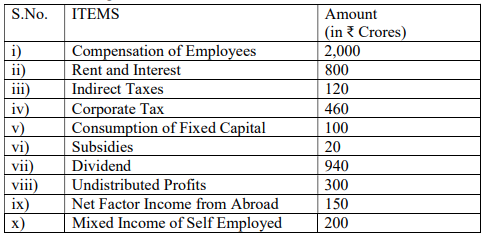# a) From the following data calculate the value of Domestic Income: b) Distinguish between ‘Value of Output’ and ‘Value Added’.

CBSE Sample Question Paper, Class 12 ECONOMICS Term 2 Question - a) From the following data calculate the value of Domestic Income: b) Distinguish between ‘Value of Output’ and ‘Value Added’.## a) From the following data calculate the value of Domestic Income: